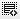## How to calculate Internal Rate of Return0
How to calculate IRR using microsoft excel..0

Please find below from MS Help section

IRR(values, [guess])
The IRR function syntax has the following arguments:

Values Required. An array or a reference to cells that contain numbers for which you want to calculate the internal rate of return.
Values must contain at least one positive value and one negative value to calculate the internal rate of return.
IRR uses the order of values to interpret the order of cash flows. Be sure to enter your payment and income values in the sequence you want.
If an array or reference argument contains text, logical values, or empty cells, those values are ignored.
Guess Optional. A number that you guess is close to the result of IRR.
Microsoft Excel uses an iterative technique for calculating IRR. Starting with guess, IRR cycles through the calculation until the result is accurate within 0.00001 percent. If IRR can't find a result that works after 20 tries, the #NUM! error value is returned.
In most cases you do not need to provide guess for the IRR calculation. If guess is omitted, it is assumed to be 0.1 (10 percent).
If IRR gives the #NUM! error value, or if the result is not close to what you expected, try again with a different value for guess.

Click on thisicon to add code snippet.